|

# 陈益峰:东楼头伤男人,西楼头旺财气

现在老百姓有一个普遍的说法，买房子风水最好的位置应该是楼房东头的位置，开发商也顺势把东头的价格提得很高，大家更是趋之若鹜，以为楼房东头风水最好，基本快形成社会的共识。实际上陈益峰师傅认为这个观点是错误的，很多人说东楼头和江景房的风水特别好，这是开发商和一些风水外行，有意无意在误导百姓的风水观念，东楼头的住宅的户型和风水位置常常是最差的。切记：常识不等于风水，共识不等于吉祥。陈益峰是当代风水名家，在风水行业具有很高的造诣，在国内外风水界有很高的知名度，是中国大陆具有正规传承的著名风水师傅，他的风水技术继承了正统的风水技术，完全脱离了鬼神迷信学说，以中国的阴阳五行理论为基础，着重研究地理地势的吉凶规律，采用大数据的手法归纳总结风水吉凶，寻找风水中的共性、普遍规律。

陈益峰师傅是当代大陆第一位成功将风水技术，成功推向海外市场的风水师，先后前往德国、波兰、澳大利亚、新加坡、日本、文莱、马来西亚等国家传播风水学说，其精湛的的技艺得到国内外广大风水爱好者的高度评价，在风水行业树立了崇高的威望。陈师傅在风水行业具有难得的实事求是的作风，具有高度的专业研究的精神，正是这股精神，感染和引领了无数风水同行，造福了无数风水家庭。

陈师傅认为住宅的东头，尤其是小区的东头常常风水很不好，主要原因就是这个位置的东边常常空旷，破坏男人的运势。别东头的户型是东户户型，东户的户型厕所常常在东边，对家中的儿子有损伤。厨房在西北角，家中男人短寿，婚姻感情不顺，在单位与领导关系不好。

1、青龙白虎

一般情况下，房子南北向，由房子里面向门口看出去，您的左侧即为龙边，右侧即为虎边，而前面为朱雀，又称明堂，屋后为玄武。要记住，房子不论坐向，永远都是东面为青龙方，西面为白虎方，南面为朱雀方，北面为玄武方，因为四兽是天上的星宿演绎过来的。2、二十八宿

古代中国天文学家先后选择了黄道赤道附近的二十八个星宿作为坐标。因为它们环列在日、月、五星的四方，很像日、月、五星栖宿的场所，所以称作二十八宿。又将其按方位及季节和四象，分为东、南、西、北四宫，每宫七宿，分别将各宫所属七宿连缀想象为一种动物，以为是“天之四灵，以正四方”。北方七宿即北宫玄武七宿，东方七宿即东宫苍龙七宿，南方七宿即南宫朱雀七宿。西方七宿即西宫白虎七宿。

东方苍龙七宿：角、亢、氐(dī)、房、心、尾、箕(jī);

北方玄武七宿：斗(dǒu)、牛、女、虚、危、室、壁;

西方白虎七宿：奎、娄(lóu)、胃、昴(mǎo)、毕、觜(zī)、参(shēn);

南方朱雀七宿：井、鬼、柳、星、张、翼、轸(zhěn)。

3、龙虎缺一边，夫妇不齐美

所谓龙虎缺一边，意思是说龙边或虎边其中有一边，没有与其它的建物相邻。如图A屋即龙边缺一边，简称龙边空，C屋即虎边缺一边，简称虎边空，而B屋因为龙、虎边皆紧邻其它房子，所以B屋两边都不缺。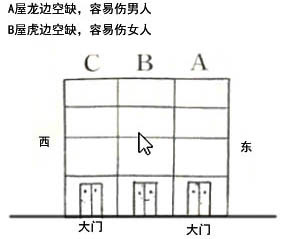空的定义在于没有其它建物或树、竹林等来覆盖遮挡。

龙边在阳宅学中，代表男性，而虎边代表女性，所以当龙边空时会造成宅中男性的身体、运势、事业各方面，每下愈况。反之，如果虎边空，则宅中女性的身体、运势、事业等会越来越差。

如果是公司或店面，龙边空会造成老板自信心不足，员工不服从命令，客户百般刁难。若虎边空，则不利女性职员，会无心上班，在公司待不住。

只要龙边为空地，没有紧邻其它建物，就称为龙边空，就会有上述之凶应，其实不然。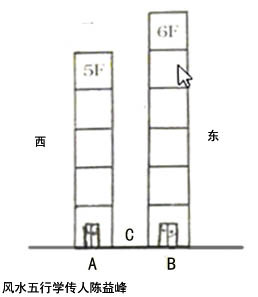如图中之A屋5层楼，B屋6层楼，A与B之间的距离-C为一层楼高的距离，则A屋的龙边为有靠(不空)，但B屋之虎边仅5楼以下有靠，6楼为空。

屋后空也会有凶应，会造成贵人不现，犯小人，受亲朋连累，破财，失和，生女的机率大于生男。

龙边或虎边空者，风水吉凶感应较慢，通常要住三年以上，才会有感应，住越久感应越严重，如家中有神位者，感应会越快。

4、楼房东头一般伤男人

一般楼房住宅不要购买最东头的位置，尤其是东面没有建筑，典型的龙边空旷，久住男人地位低下，事业不振，夫妻失和，儿子不听话等。楼房最西头的位置，尤其是西面没有建筑，一般旺财气，旺男人，旺儿子，但是女人多身体不好，甚至短寿。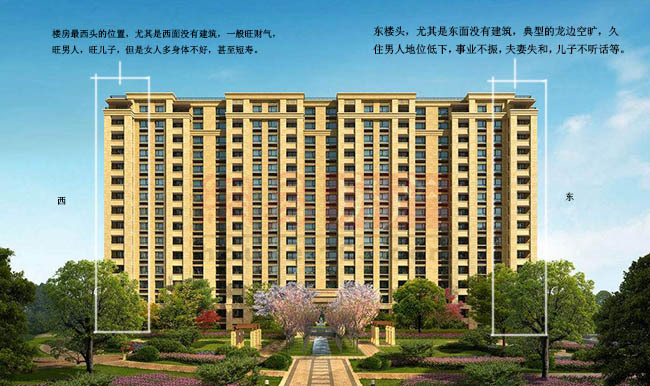5、东户的户型风水也有问题

东楼头的房子基本都是东户的户型，关于东为上的说法，风水没有吉凶根据，但是大多数东户常常风水不好，主要原因就是厕所在东面伤长子，厨房在西北角伤男人，伤寿命，伤事业。

(1)、东厕伤长子

东方后天八卦为震卦，先天八卦为离卦，震卦主长子、主事业、主贵人，离卦主中女、眼睛、心脏、血液等疾病。东方有厕所的家庭伤儿子，视力下降，偶尔也有精神疾病。东为震卦，震卦是长子也就是大儿子，伤长子的最多，无男丁之家的口舌多，呼吸系统疾病跑不了，东面有卫生间久住最轻的也要近视眼，视力下降。东卫生间住久了还会容易有足疾、肝经之疾、惊恐不安、精神病、多动症、羊癫病、精神衰弱、神经过敏、妇科病、疼痛性症、咳嗽、咽喉病症。对于城市楼房住宅来讲，一般厕所在西侧比东侧旺财气，旺儿子，旺老公，出仁义善良之辈。西户的住宅厕所一般都在西侧。所以陈益峰师傅强烈建议购买西户，如果户主是属兔、鸡的人更加倒霉。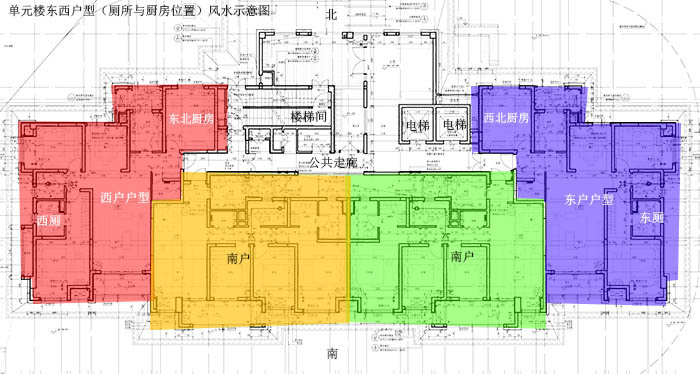(2)、厨房西北角

一般情况下，东户的户型，厨房在西北角占到百分之九十以上，厨房西北角，风水上为第一大忌讳，名叫火烧天门。古人认为，“火烧天门”这种格局会损害家庭中男主人或男性长者的运气和健康，易导致男主人脾气暴躁、引发呼吸系统、脑部等疾病。而且家中易出忤逆儿女，如果户主是属龙、蛇、猪、狗的人，最为倒霉。

实际上家中的西北方位见到炉灶、高物、大柜子等，均是火烧天门。因为在风水中，西北方为乾位，即天门，乾卦，是家中父亲角色的人的位置，又是升官发财的关键位置，宜生不宜克，生则有利，克则必凶。乾卦五行属金，最怕火来克，而炉灶正是火旺之物，位于西北便是重火克金的格局，因此称为火烧天门。实际上凡是在西北方位见到阳性火旺的物体，如红色的大柜子、高山、高塔、变压器、桥梁、大树、消防队、部队大院、寺庙、公安局、检察院、法院等等，都以火论，都视为广义的“火烧天门”。

总而言之，单元楼或者塔楼的东边户风水常常出问题，价格也是常常最贵的，也是最容易出手的，陈师傅认为西户相对东户风水更好，西楼头相对东楼头风水更好，西户一般提升人的福气，东户一般降低人的福气。（记者/ 刘一婷）

`声明：本文由入驻焦点开放平台的作者撰写，除焦点官方账号外，观点仅代表作者本人，不代表焦点立场错误信息举报电话： 400-099-0099，邮箱：jubao@vip.sohu.com，或点此进行意见反馈，或点此进行举报投诉。`A B C D E F G H J K L M N P Q R S T W X Y Z
A - B - C - D - E
• A
• 鞍山
• 安庆
• 安阳
• 安顺
• 安康
• 澳门
• B
• 北京
• 保定
• 包头
• 巴彦淖尔
• 本溪
• 蚌埠
• 亳州
• 滨州
• 北海
• 百色
• 巴中
• 毕节
• 保山
• 宝鸡
• 白银
• 巴州
• C
• 承德
• 沧州
• 长治
• 赤峰
• 朝阳
• 长春
• 常州
• 滁州
• 池州
• 长沙
• 常德
• 郴州
• 潮州
• 崇左
• 重庆
• 成都
• 楚雄
• 昌都
• 慈溪
• 常熟
• D
• 大同
• 大连
• 丹东
• 大庆
• 东营
• 德州
• 东莞
• 德阳
• 达州
• 大理
• 德宏
• 定西
• 儋州
• 东平
• E
• 鄂尔多斯
• 鄂州
• 恩施
F - G - H - I - J
• F
• 抚顺
• 阜新
• 阜阳
• 福州
• 抚州
• 佛山
• 防城港
• G
• 赣州
• 广州
• 桂林
• 贵港
• 广元
• 广安
• 贵阳
• 固原
• H
• 邯郸
• 衡水
• 呼和浩特
• 呼伦贝尔
• 葫芦岛
• 哈尔滨
• 黑河
• 淮安
• 杭州
• 湖州
• 合肥
• 淮南
• 淮北
• 黄山
• 菏泽
• 鹤壁
• 黄石
• 黄冈
• 衡阳
• 怀化
• 惠州
• 河源
• 贺州
• 河池
• 海口
• 红河
• 汉中
• 海东
• 怀来
• I
• J
• 晋中
• 锦州
• 吉林
• 鸡西
• 佳木斯
• 嘉兴
• 金华
• 景德镇
• 九江
• 吉安
• 济南
• 济宁
• 焦作
• 荆门
• 荆州
• 江门
• 揭阳
• 金昌
• 酒泉
• 嘉峪关
K - L - M - N - P
• K
• 开封
• 昆明
• 昆山
• L
• 廊坊
• 临汾
• 辽阳
• 连云港
• 丽水
• 六安
• 龙岩
• 莱芜
• 临沂
• 聊城
• 洛阳
• 漯河
• 娄底
• 柳州
• 来宾
• 泸州
• 乐山
• 六盘水
• 丽江
• 临沧
• 拉萨
• 林芝
• 兰州
• 陇南
• M
• 牡丹江
• 马鞍山
• 茂名
• 梅州
• 绵阳
• 眉山
• N
• 南京
• 南通
• 宁波
• 南平
• 宁德
• 南昌
• 南阳
• 南宁
• 内江
• 南充
• P
• 盘锦
• 莆田
• 平顶山
• 濮阳
• 攀枝花
• 普洱
• 平凉
Q - R - S - T - W
• Q
• 秦皇岛
• 齐齐哈尔
• 衢州
• 泉州
• 青岛
• 清远
• 钦州
• 黔南
• 曲靖
• 庆阳
• R
• 日照
• 日喀则
• S
• 石家庄
• 沈阳
• 双鸭山
• 绥化
• 上海
• 苏州
• 宿迁
• 绍兴
• 宿州
• 三明
• 上饶
• 三门峡
• 商丘
• 十堰
• 随州
• 邵阳
• 韶关
• 深圳
• 汕头
• 汕尾
• 三亚
• 三沙
• 遂宁
• 山南
• 商洛
• 石嘴山
• T
• 天津
• 唐山
• 太原
• 通辽
• 铁岭
• 泰州
• 台州
• 铜陵
• 泰安
• 铜仁
• 铜川
• 天水
• 天门
• W
• 乌海
• 乌兰察布
• 无锡
• 温州
• 芜湖
• 潍坊
• 威海
• 武汉
• 梧州
• 渭南
• 武威
• 吴忠
• 乌鲁木齐
X - Y - Z
• X
• 邢台
• 徐州
• 宣城
• 厦门
• 新乡
• 许昌
• 信阳
• 襄阳
• 孝感
• 咸宁
• 湘潭
• 湘西
• 西双版纳
• 西安
• 咸阳
• 西宁
• 仙桃
• 西昌
• Y
• 运城
• 营口
• 盐城
• 扬州
• 鹰潭
• 宜春
• 烟台
• 宜昌
• 岳阳
• 益阳
• 永州
• 阳江
• 云浮
• 玉林
• 宜宾
• 雅安
• 玉溪
• 延安
• 榆林
• 银川
• Z
• 张家口
• 镇江
• 舟山
• 漳州
• 淄博
• 枣庄
• 郑州
• 周口
• 驻马店
• 株洲
• 张家界
• 珠海
• 湛江
• 肇庆
• 中山
• 自贡
• 资阳
• 遵义
• 昭通
• 张掖
• 中卫

1室1厅1厨1卫1阳台

1
2
3
4
5

0
1
2

1

1

0
1
2
3报名成功，资料已提交审核A B C D E F G H J K L M N P Q R S T W X Y Z
A - B - C - D - E
• A
• 鞍山
• 安庆
• 安阳
• 安顺
• 安康
• 澳门
• B
• 北京
• 保定
• 包头
• 巴彦淖尔
• 本溪
• 蚌埠
• 亳州
• 滨州
• 北海
• 百色
• 巴中
• 毕节
• 保山
• 宝鸡
• 白银
• 巴州
• C
• 承德
• 沧州
• 长治
• 赤峰
• 朝阳
• 长春
• 常州
• 滁州
• 池州
• 长沙
• 常德
• 郴州
• 潮州
• 崇左
• 重庆
• 成都
• 楚雄
• 昌都
• 慈溪
• 常熟
• D
• 大同
• 大连
• 丹东
• 大庆
• 东营
• 德州
• 东莞
• 德阳
• 达州
• 大理
• 德宏
• 定西
• 儋州
• 东平
• E
• 鄂尔多斯
• 鄂州
• 恩施
F - G - H - I - J
• F
• 抚顺
• 阜新
• 阜阳
• 福州
• 抚州
• 佛山
• 防城港
• G
• 赣州
• 广州
• 桂林
• 贵港
• 广元
• 广安
• 贵阳
• 固原
• H
• 邯郸
• 衡水
• 呼和浩特
• 呼伦贝尔
• 葫芦岛
• 哈尔滨
• 黑河
• 淮安
• 杭州
• 湖州
• 合肥
• 淮南
• 淮北
• 黄山
• 菏泽
• 鹤壁
• 黄石
• 黄冈
• 衡阳
• 怀化
• 惠州
• 河源
• 贺州
• 河池
• 海口
• 红河
• 汉中
• 海东
• 怀来
• I
• J
• 晋中
• 锦州
• 吉林
• 鸡西
• 佳木斯
• 嘉兴
• 金华
• 景德镇
• 九江
• 吉安
• 济南
• 济宁
• 焦作
• 荆门
• 荆州
• 江门
• 揭阳
• 金昌
• 酒泉
• 嘉峪关
K - L - M - N - P
• K
• 开封
• 昆明
• 昆山
• L
• 廊坊
• 临汾
• 辽阳
• 连云港
• 丽水
• 六安
• 龙岩
• 莱芜
• 临沂
• 聊城
• 洛阳
• 漯河
• 娄底
• 柳州
• 来宾
• 泸州
• 乐山
• 六盘水
• 丽江
• 临沧
• 拉萨
• 林芝
• 兰州
• 陇南
• M
• 牡丹江
• 马鞍山
• 茂名
• 梅州
• 绵阳
• 眉山
• N
• 南京
• 南通
• 宁波
• 南平
• 宁德
• 南昌
• 南阳
• 南宁
• 内江
• 南充
• P
• 盘锦
• 莆田
• 平顶山
• 濮阳
• 攀枝花
• 普洱
• 平凉
Q - R - S - T - W
• Q
• 秦皇岛
• 齐齐哈尔
• 衢州
• 泉州
• 青岛
• 清远
• 钦州
• 黔南
• 曲靖
• 庆阳
• R
• 日照
• 日喀则
• S
• 石家庄
• 沈阳
• 双鸭山
• 绥化
• 上海
• 苏州
• 宿迁
• 绍兴
• 宿州
• 三明
• 上饶
• 三门峡
• 商丘
• 十堰
• 随州
• 邵阳
• 韶关
• 深圳
• 汕头
• 汕尾
• 三亚
• 三沙
• 遂宁
• 山南
• 商洛
• 石嘴山
• T
• 天津
• 唐山
• 太原
• 通辽
• 铁岭
• 泰州
• 台州
• 铜陵
• 泰安
• 铜仁
• 铜川
• 天水
• 天门
• W
• 乌海
• 乌兰察布
• 无锡
• 温州
• 芜湖
• 潍坊
• 威海
• 武汉
• 梧州
• 渭南
• 武威
• 吴忠
• 乌鲁木齐
X - Y - Z
• X
• 邢台
• 徐州
• 宣城
• 厦门
• 新乡
• 许昌
• 信阳
• 襄阳
• 孝感
• 咸宁
• 湘潭
• 湘西
• 西双版纳
• 西安
• 咸阳
• 西宁
• 仙桃
• 西昌
• Y
• 运城
• 营口
• 盐城
• 扬州
• 鹰潭
• 宜春
• 烟台
• 宜昌
• 岳阳
• 益阳
• 永州
• 阳江
• 云浮
• 玉林
• 宜宾
• 雅安
• 玉溪
• 延安
• 榆林
• 银川
• Z
• 张家口
• 镇江
• 舟山
• 漳州
• 淄博
• 枣庄
• 郑州
• 周口
• 驻马店
• 株洲
• 张家界
• 珠海
• 湛江
• 肇庆
• 中山
• 自贡
• 资阳
• 遵义
• 昭通
• 张掖
• 中卫• 手机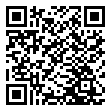• 分享
• 设计
免费设计
• 计算器
装修计算器
• 入驻
合作入驻
• 联系
联系我们
• 置顶
返回顶部